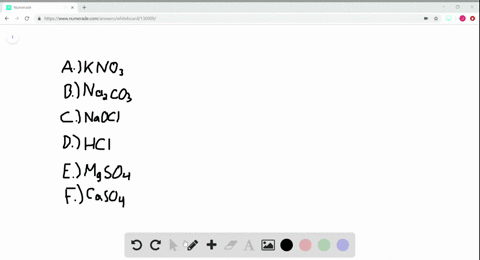### Many familiar substances have common, unsystemati…

03:10Manhattan College
Problem 111

Give the chemical names of each of the following familiar compounds:$(\mathbf{a})$$\mathrm{NaCl}( table salt ) (\mathbf{b})$$\mathrm{NaHCO}_{3}$ (baking soda),$(\mathbf{c}) \mathrm{NaOCl}$ (in many branches)$(\mathbf{d})$ $\mathrm{NaOH}($ caustic soda $)$ $(\mathbf{e})$ $\left(\mathrm{NH}_{4}\right)_{2} \mathrm{CO}_{3}($ smelling salts $)$$(\mathbf{f})$$\mathrm{CaSO}_{4}$ (plaster of Paris).

## Discussion

You must be signed in to discuss.

## Video Transcript

problem 1 11 except to give the chemical name to each of the familiar compounds and a CEO. Oh, we just table salt and h c 03 This is known as baking soda and a O. C. O. Which is used in a block of bleachers. Sorry, Emmett. Many branches I should say Hey! And a ohh. Which is caustic soda and h for to see Oh, three just smelling salts and C A s 04 which is the Glasser of Paris. So remember when we named these things the metal comes first and then the non metal so we have sodium, and then we have chlorine. But remember, it's eye on a compound because it's composed of a metal and non metal. It's a sodium chloride. All right, here we have sodium, and we have a t 03 which knows bicarbonate. Here we have Excuse me. So we have sodium hypo folklore chloride because hypo chlorate is o. C E o. Next year, we have sodium hydroxide, so hydroxide is o H minus. And remember, you have an A plus for our sodium Zagato. So which minds is going to be our non metal or partly atomic. I am here we have and h four, which is gonna be ammonium. Um, when we have to. I have a compound made out of two non metals meadows. We used the positively charged one first and then we use the negative charge. So we have ammonium. And then after that, we have what is known as carbonates. Now, next we have calcium, which is C a and then we are going to have I have so faint. Eso eso four This is very strange folk. So we're just remember the name and convince.